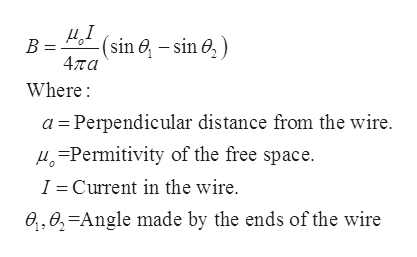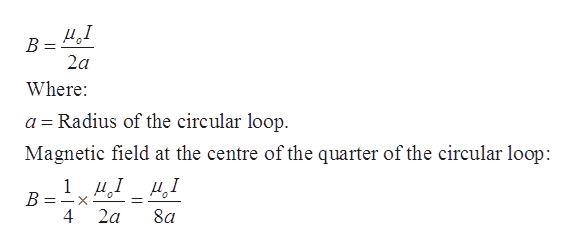# a

Question

An “infinitely” long wire is bent into the shape seen below.  It carries a current of 14 Amps in the direction given by the arrow heads.  The curved part of the wire is a quarter-circle of radius a = 3.0 cm.

a)  Determine the direction of the total magnetic field produced by the wire at the origin. Explain your answer in words.

b)  Calculate the magnitude of the total magnetic field produced by the wire at the origin.

check_circle

Step 1

Given values:

Current in the wire, I = 14 A

Radius of the curved part of the wire, a = 3.0 cm = 0.03 m

The magnetic field due to straight wire can be given by the formula:help_outlineImage Transcriptionclose(sin e -sin ) B = 4πα Where a Perpendicular distance from the wire. Permity of the free space. Current in the wire. 1 e,0,-Angle made by the ends of the wire fullscreen
Step 2

The magnetic field at the centre of current carrying loop:help_outlineImage TranscriptioncloseД.1 В 2a Where Radius of the circular loop a Magnetic field at the centre of the quarter of the circular loop: 1, Д.1 Д,1 =- 2a 4 8a fullscreen
Step 3

(a)

From the right-hand thumb rule, the direction of the total magnetic field at the origin would be point into the page.

...

### Want to see the full answer?

See Solution

#### Want to see this answer and more?

Solutions are written by subject experts who are available 24/7. Questions are typically answered within 1 hour.*

See Solution
*Response times may vary by subject and question.
Tagged in

### Other